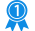15010
2585

# .NET Matrix Library (2.5.5000.811) Free Download

.NET Matrix Library 2.5.5000.811 Description:
The Bluebit .NET Matrix Library provides classes for object-oriented linear algebra in the .NET platform. It can be used to solve systems of simultaneous linear equations, least-squares solutions of linear systems of equations, eigenvalues and eigenvectors problems, and singular value problems. Also provided are the associated matrix factorizations such as Eigen, LQ, LU, Cholesky, QR, SVD. The above functionality is present for both real and complex matrices. Two analogous sets of classes are provided for real and complex matrices, vectors and factorizations. While exposing an easy to use and powerful interface, the Bluebit .NET Matrix Library does not sacrifice any performance. Highly optimized BLAS and the standard LAPACK routines are used within the library and provide fast execution and accurate calculations. The Bluebit .NET Matrix Library has been developed as a mixed mode C++ project, combining together managed and unmanaged code and delivering the best of both worlds; the speed of native C++ code and the feature-rich and easy to use environment of the .NET Framework.

Keywords : matrix,vector,math,eigenvalues,eigenvalue,eigenvectors,eigenvector,qr,cholesky,lu,decomposition,svd,net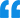.NET Matrix Library: The Bluebit .NET Matrix Library provides classes for object-oriented algebra. Solves systems of simultaneous linear equations, least-squares solutions of linear systems of equations, eigenvalues and eigenvectors problems, and singular value problems.
Publisher: Bluebit Software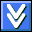Download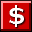Buy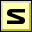Screenshot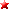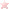Rate it
Category: General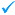Publisher: Bluebit SoftwareMore titles >>
Last Updated: 02/13/2019
Requirements: .NET Framework 1.1